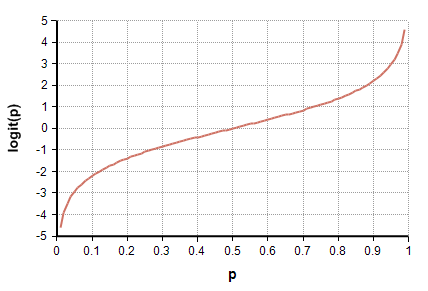# Logit

## Logit(p)

The Logit function is equal to

$\displaystyle{ Logit(p) = \ln\left( p\over{1-p} \right) }$

where $\displaystyle{ 0\lt p\lt 1 }$. The logit is also called the log-odds of a probability $\displaystyle{ p }$.The inverse of the Logit function is Sigmoid(x) (before Analytica 4.5, the function InvLogit was used, defined in the Generalized Regression library). The logit function is sometimes called the log-odds function.

## Complex numbers

When p < 0 or p > 1, a warning is issued and if the warning is ignored, the result is NaN. This is the case unless you have enabled complex numbers. If you have set the system variable EnableComplexNumbers to 1, then no warning is issued and Logit(p) returns a complex number.

## History

Logit was introduced as a built-in function in Analytica 4.5. Before that, it was supplied as a function in the Generalized Regression library.